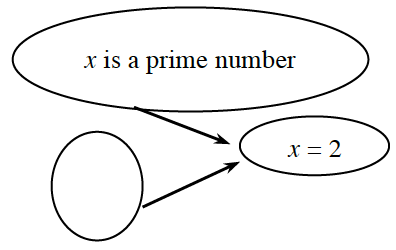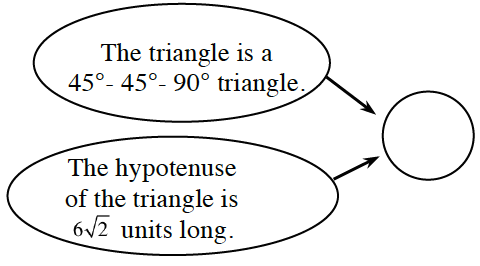### Home > CCG > Chapter 5 > Lesson 5.2.1 > Problem5-56

5-56.

Fill in the blank ovals below so that each flowchart is correct.

1.The fact is $x$ is a prime number so the conclusion is $x=2$, so $x=2$ is a prime number.
What is another fact you can say that would still give $x=2$ as a conclusion.
Put that in the blank oval.

$x$ is even, or $x$ is less than $3$ are possible answers, because these fact lead to the conclusion $x=2$.

1.What conclusion can you make about the legs of the triangle?